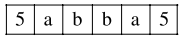# A number is called a palindrome

Question:

A number is called a palindrome if it reads the same backward as well as forward. For example 285582 is a six digit palindrome. The number of six digit palindromes, which are divisible by 55 , is

Solution:It is always divisible by 5 and 11 .

So, required number $=10 \times 10=100$